# Point Symmetry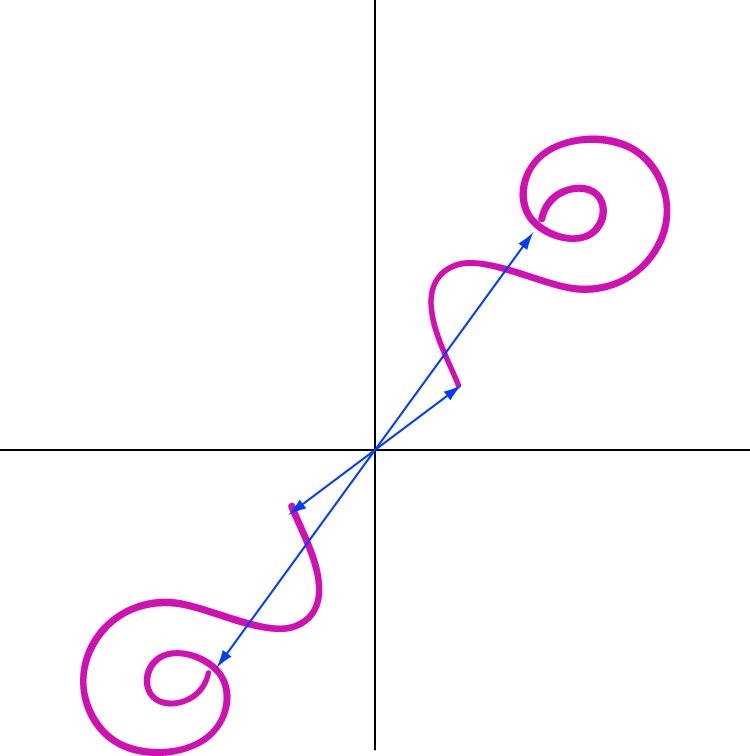An object has point symmetry if each of its points has a matching point that is the same distance, but in the opposite direction from some fixed central point called the origin.

Objects that have point symmetry look the same right way up as they do upside down. They also look the same from the left and the right, or from any other pair of opposite directions.

Another term for point symmetry is rotational symmetry of order 2.

## Examples of Things with Point Symmetry

Geometric shapes: some geometric shapes like rectangles and this parallelogram have point symmetry.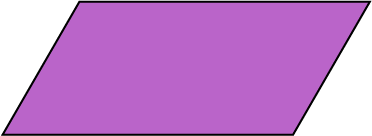Ladders have point symmetry, provided that the distance from the bottom of ladder to the bottom rung is the same as the distance from the top rung to the top of the ladder.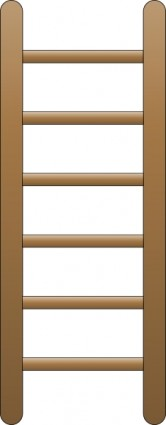Propellors with two blades: many propellors, like the one on this toy plane, have point symmetry as they have two blades, so they look the same from the top and the bottom, or the left and the right.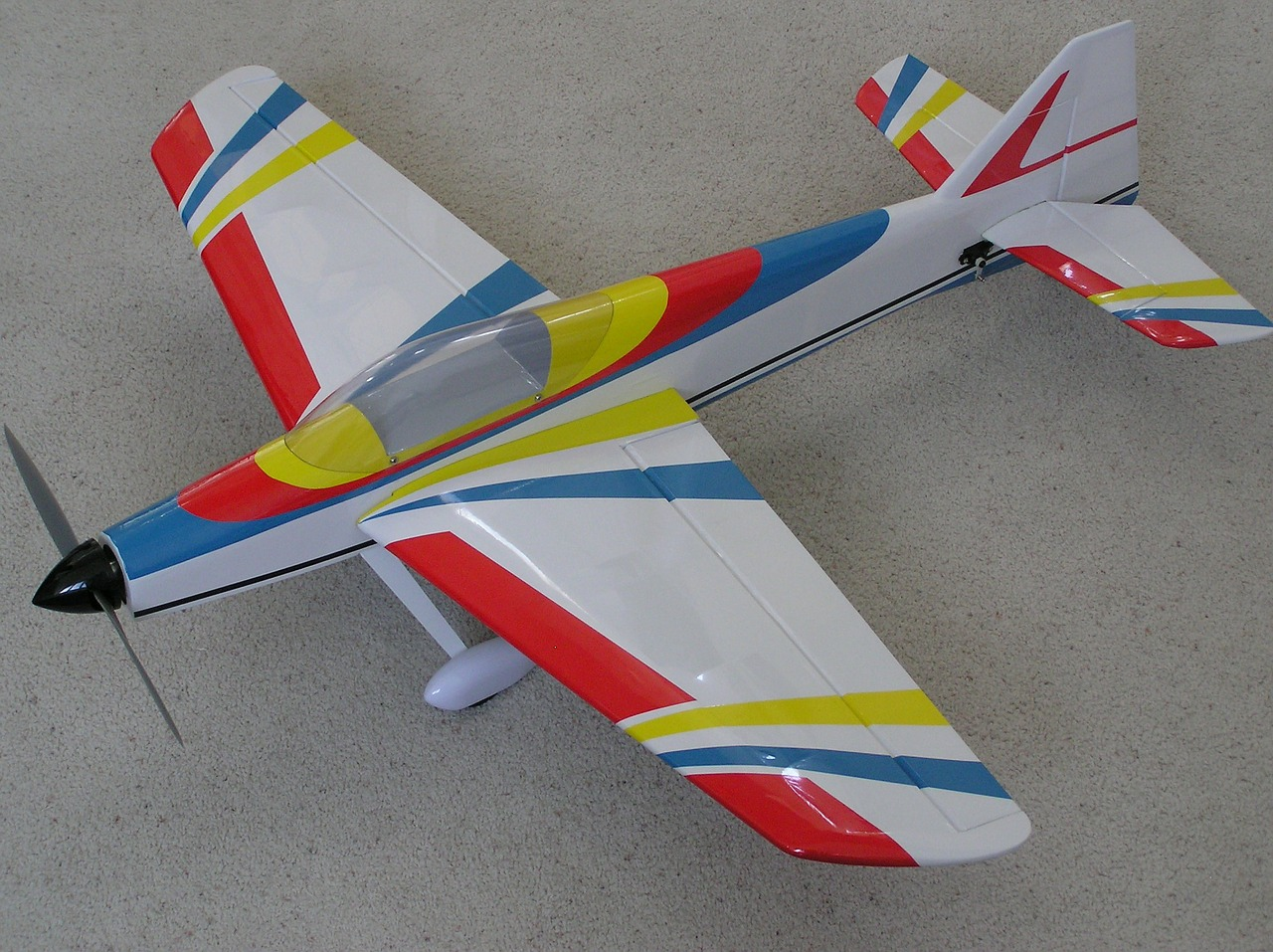A few flags have point symmetry. For example, this UK flag has point symmetry, as does the Eureka flag. However, the Australian and New Zealand flags do not have point symmetry.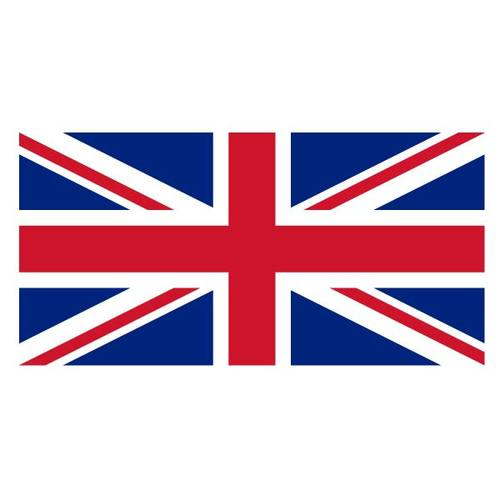Many playing cards have point symmetry. They look the same, no matter what direction you look at them from: left or right, up or down, at a $45^\circ$ angle or a $225^\circ$ angle, at a $30^\circ$ angle or at a $210^\circ$ angle, and so on.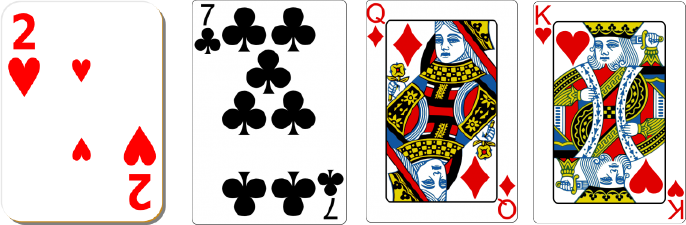Some letters have point symmetry. Here are some of them. See if you can think of any more.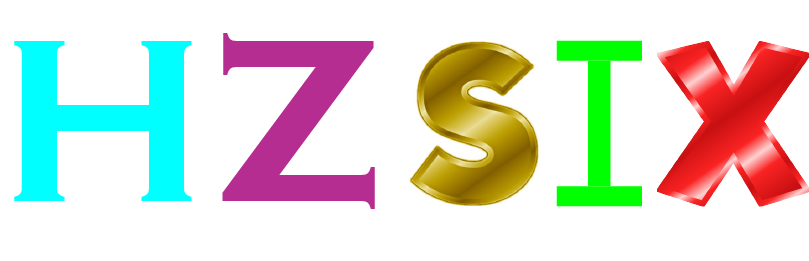If you forget about colouring half of it black and the other half white, then the yin-yang symbol has point symmetry.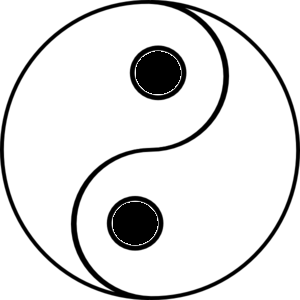### Conclusion

Many objects have point symmetry: they look the same when you look at them in opposite directions. It might be easier to remember that point symmetry is the same as rotational symmetry of order 2: so the object looks exactly like itself twice in a full rotation. Why don't you see if you can find some other objects with point symmetry? To get started, have a think about some of the symbols we use in mathematics, or some geometric shapes. It's fun trying to spot symmetries in objects!

### Description

This mini book covers the core of Math for Foundation, Grade 1 and Grade 2 mathematics including

1. Numbers
3. Subtraction
4. Division
5. Algebra
6. Geometry
7. Data
8. Estimation
9. Probability/Chance
10. Measurement
11. Time
12. Money
13. and much more

This material is provided free of cost for Parent looking for some tricks for their Prekinder, Kinder, Prep, Year 1 and Year 2 children

### Learning Objectives

These lessons are for kids aged 4-8 with the core objective to expose their brains to concepts of addition, subtraction, division, algebra and much more.

Author: Subject Coach
You must be logged in as Student to ask a Question.